• 在TD-LTE系统中，Turbo码...在高斯白噪声信道（AWGN）环境下，对各种Turbo译码算法性能进行了仿真比较，并对提出的简化译码算法在TMS320C64xDSP中进行实现。译码程序在CCS3.3的运行结果表明了该方案的实时性和有效性。
• 分析了两种分块并行 Turbo译码算法,并提出了一种新型的混合算法。研究表明,与现有的两种算法相比,混合算法融合了两者的优点,虽多消耗了少量资源,但是明显改善了译码性能。
• Turbo码是现在非常流行的编码方法，其优异性能主要来源于迭代的译码算法，本文分析对比了几种经典的算法，并对每种算法的资源消耗情况给予了定量的计算
• turbo译码器，用C++实现的，代码完整，性能优异，可以直接用
• turbo译码Logmap算法和sova算法 2.部分程序： function L_all = sova(rec_s, g, L_a, ind_dec) % This function implememts Soft Output Viterbi Algorithm in trace back mode % Input: % rec_s: scaled ...
1.问题描述：
turbo译码Logmap算法和sova算法
2.部分程序：

function L_all = sova(rec_s, g, L_a, ind_dec)  % This function implememts Soft Output Viterbi Algorithm in trace back mode  % Input:  %       rec_s: scaled received bits. rec_s(k) = 0.5 * L_c(k) * y(k)  %              L_c = 4 * a * Es/No, reliability value of the channel %              y: received bits %       g:  encoder generator matrix in binary form, g(1,:) for feedback, g(2,:) for feedforward %       L_a: a priori information about the info. bits. Extrinsic info. from the previous %             component decoder %       ind_dec: index of the component decoder.  %              =1: component decoder 1; The trellis is terminated to all zero state %                  =2: component decoder 2; The trellis is not perfectly terminated. % Output: %       L_all: log ( P(x=1|y) ) / ( P(x=-1|y) ) %
% Frame size, info. + tail bits L_total = length(L_a); [n,K] = size(g);  m = K - 1; nstates = 2^m; Infty = 1e10;
% SOVA window size. Make decision after 'delta' delay. Decide bit k when received bits % for bit (k+delta) are processed. Trace back from (k+delta) to k.  delta = 30;
% Set up the trellis defined by g. [next_out, next_state, last_out, last_state] = trellis(g);
% Initialize path metrics to -Infty for t=1:L_total+1    for state=1:nstates       path_metric(state,t) = -Infty;    end end
% Trace forward to compute all the path metrics path_metric(1,1) = 0; for t=1:L_total    y = rec_s(2*t-1:2*t);    for state=1:nstates       sym0 = last_out(state,1:2);       sym1 = last_out(state,3:4);       state0 = last_state(state,1);       state1 = last_state(state,2);       Mk0 = y*sym0' - L_a(t)/2 + path_metric(state0,t);       Mk1 = y*sym1' + L_a(t)/2 + path_metric(state1,t);              if Mk0>Mk1          path_metric(state,t+1)=Mk0;          Mdiff(state,t+1) = Mk0 - Mk1;          prev_bit(state, t+1) = 0;       else          path_metric(state,t+1)=Mk1;          Mdiff(state,t+1) = Mk1 - Mk0;          prev_bit(state,t+1) = 1;       end
end end        % For decoder 1, trace back from all zero state,  % for decoder two, trace back from the most likely state if ind_dec == 1    mlstate(L_total+1) = 1; else    mlstate(L_total+1) = find( path_metric(:,L_total+1)==max(path_metric(:,L_total+1)) ); end
% Trace back to get the estimated bits, and the most likely path for t=L_total:-1:1    est(t) = prev_bit(mlstate(t+1),t+1);    mlstate(t) = last_state(mlstate(t+1), est(t)+1); end
% Find the minimum delta that corresponds to a compitition path with different info. bit estimation.        % Give the soft output for t=1:L_total    llr = Infty;    for i=0:delta       if t+i<L_total+1          bit = 1-est(t+i);          temp_state = last_state(mlstate(t+i+1), bit+1);          for j=i-1:-1:0             bit = prev_bit(temp_state,t+j+1);             temp_state = last_state(temp_state, bit+1);          end          if bit~=est(t)              llr = min( llr,Mdiff(mlstate(t+i+1), t+i+1) );          end       end    end    L_all(t) = (2*est(t) - 1) * llr; end
function L_all = logmapo(rec_s,g,L_a,ind_dec)
% Log_MAP algorithm using straightforward method to compute branch metrics % no approximation is used. % Can be simplified to Max-Log-MAP by using approximation ln(e^x+e^y) = max(x,y). % Input: rec_s: scaled received bits.  %               rec_s = 0.5 * L_c * yk = ( 2 * a * rate * Eb/N0 ) * yk %        g: code generator for the component RSC code, in binary matrix form. %        L_a: a priori info. for the current decoder,  %               scrambled version of extrinsic Inftyo. of the previous decoder. %        ind_dec: index of decoder. Either 1 or 2.  %               Encoder 1 is assumed to be terminated, while encoder 2 is open. % % Output: L_all: log-likelihood ratio of the symbols. Complete information.
% Total number of bits: Inftyo. + tail L_total = length(rec_s)/2; [n,K] = size(g);  m = K - 1; nstates = 2^m;
% Set up the trellis [next_out, next_state, last_out, last_state] = trellis(g);
Infty = 1e10;
% Initialization of Alpha Alpha(1,1) = 0;  Alpha(1,2:nstates) = -Infty*ones(1,nstates-1);
% Initialization of Beta if ind_dec==1    Beta(L_total,1) = 0;    Beta(L_total,2:nstates) = -Infty*ones(1,nstates-1);  elseif ind_dec==2    Beta(L_total,1:nstates) = zeros(1,nstates); else    fprintf('ind_dec is limited to 1 and 2!\n'); end
% Trace forward, compute Alpha for k = 2:L_total+1     for state2 = 1:nstates       gamma = -Infty*ones(1,nstates);       gamma(last_state(state2,1)) = (-rec_s(2*k-3)+rec_s(2*k-2)*last_out(state2,2))....            -log(1+exp(L_a(k-1)));       gamma(last_state(state2,2)) = (rec_s(2*k-3)+rec_s(2*k-2)*last_out(state2,4))....            +L_a(k-1)-log(1+exp(L_a(k-1)));
if(sum(exp(gamma+Alpha(k-1,:)))<1e-300)          Alpha(k,state2)=-Infty;       else          Alpha(k,state2) = log( sum( exp( gamma+Alpha(k-1,:) ) ) );         end        end     tempmax(k) = max(Alpha(k,:));     Alpha(k,:) = Alpha(k,:) - tempmax(k); end
% Trace backward, compute Beta for k = L_total-1:-1:1   for state1 = 1:nstates      gamma = -Infty*ones(1,nstates);      gamma(next_state(state1,1)) = (-rec_s(2*k+1)+rec_s(2*k+2)*next_out(state1,2))....            -log(1+exp(L_a(k+1)));      gamma(next_state(state1,2)) = (rec_s(2*k+1)+rec_s(2*k+2)*next_out(state1,4))....            +L_a(k+1)-log(1+exp(L_a(k+1)));      if(sum(exp(gamma+Beta(k+1,:)))<1e-300)         Beta(k,state1)=-Infty;      else         Beta(k,state1) = log(sum(exp(gamma+Beta(k+1,:))));      end      end   Beta(k,:) = Beta(k,:) - tempmax(k+1); end
% Compute the soft output, log-likelihood ratio of symbols in the frame for k = 1:L_total   for state2 = 1:nstates      gamma0 = (-rec_s(2*k-1)+rec_s(2*k)*last_out(state2,2))....            -log(1+exp(L_a(k)));      gamma1 = (rec_s(2*k-1)+rec_s(2*k)*last_out(state2,4))...            +L_a(k)-log(1+exp(L_a(k)));      temp0(state2) = exp(gamma0 + Alpha(k,last_state(state2,1)) + Beta(k,state2));      temp1(state2) = exp(gamma1 + Alpha(k,last_state(state2,2)) + Beta(k,state2));   end   L_all(k) = log(sum(temp1)) - log(sum(temp0)); end

3.仿真结论：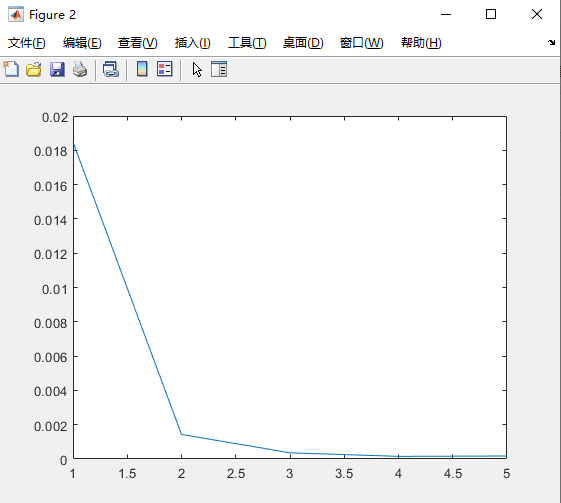D36
展开全文matlab
• turbo全套资源urb全套 吴雨霏理论代码 ccsds 不同译码算法
• 针对LTE系统中的Turbo译码算法进行了研究，在Max-Log-MAP和Log-MAP算法的基础上提出了一种改进算法。理论分析和仿真结果表明，这种改进算法的性能有进一步提升，并且更加易于硬件实现，具有较低的复杂度。
• 为此,提出一种基于修正函数线性拟合的Turbo译码算法,该算法针对不同刻度区间采用不同的拟合参数。实验仿真表明,该算法与现有算法比较,能够达到Log-MAP算法的译码性能,且避免了Log-MAP的大量运算,从而在保证较好译码...
• 分析了传统Chase 算法中寻找 竞争码字对译码复杂度的影响，在此基础上提出了两种新的简化译码算法，省去了寻找竞争码字的过程。仿 真结果表明，简化算法在基本保持传统Chase 算法译码性能的基础上，降低了译码...
• 针对Turbo乘积码（TPC）译码复杂度高、运算量大的缺点，分析了一种改进的TPC译码算法。该算法以Chase迭代算法为基础，通过对错误图样重新排序产生新的测试序列，其伴随式可从前次伴随式的基础上修正一位得到，大大...
• 为了提高传统迭代译码的Turbo译码算法，本文把Turbo码和CRC看成一个级联码，研究利用CRC的冗余来增强Turbo译码性能。由于Turbo码的分量码是卷积码，所以采用基于List Viterbi（LVA）的算法进行Turbo译码。 一、主要...
为了提高传统迭代译码的Turbo译码算法，本文把Turbo码和CRC看成一个级联码，研究利用CRC的冗余来增强Turbo译码性能。由于Turbo码的分量码是卷积码，所以采用基于List Viterbi（LVA）的算法进行Turbo译码。
一、主要思想
Viterbi算法是一种概率译码算法，其译码是寻找使得接收序列条件概率最大的码字，是将接收的信号序列和所有可能的发射码字序列进行相关，然后从中选取似然值最大的序列。因此Viterbi算法是最大似然估计算法。Viterbi算法在码的约束度较小时，速度较快，译码器也较简单。
LVA即列表维特比译码，是一种改进的Viterbi译码算法，与传统仅产生一条最优路径不同的是，LVA算法能产生多条次优路径，通过CRC从中进行选择。主要思想是在原有的Turbo迭代译码基础上，利用迭代的软输出量对分量码即卷积码进行LVA算法，输出多条最优路径，由CRC进行错误检测和提前终止迭代获次优路径输出。LVA译码可在每次分量译码器的译码之后进行。LVA算法处理框图如下：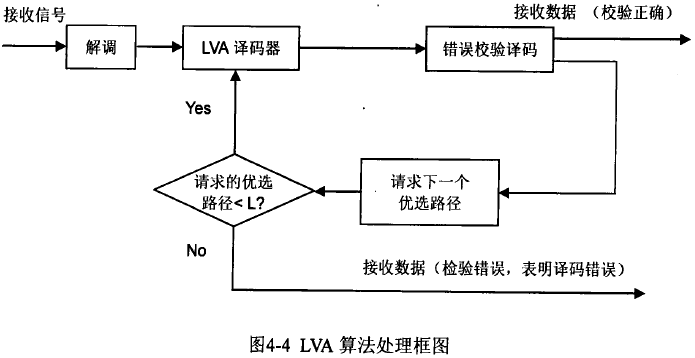LVA算法从全局优选路径中选择最有可能的L个正确路径，增大了找到正确路径的概率，代价是增加搜索过程中的计算量和路径存储量。在实际应用中，需要在带来的增益和实现的复杂性之间进行折衷。
LVA算法分为两种：并行次优路径算法(Parallel List Viterbi Algorithm，PLVA)和串行次优路径算法(Serial List Virerbi Algorithm，SLVA)。PLVA通过一次Trellis格图搜索，同时得到L个全局优选路径。SLVA是迭代获得L条路径，第L条路径由所有前L-1条路径获得，例如最优路径L=1，根据L=1得次优路径L=2，根据L=1和L=2得到L=3，依次递推。
二、PLVA算法
PLVA算法与Viterbi算法的步骤大体上相同，只是对于每个时刻的每一个状态，都有2L个路径进入，在2L个路径中选择得L个局部优选路径，当L=I时，就是Viterbi算法。PLVA算法中每个状态优选路径选取如图所示。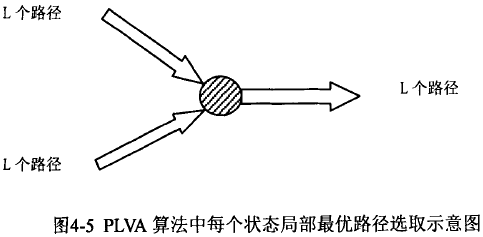PLVA算法的具体步骤如下： (1)初始化t=l时刻的路径度量和指向初始0状态的路径。
(2)当l<t<T时，对于每个时刻的每一个状态进行分别计算2个分支度量和2L个路径度量，比较选择得到L个局部优选路径，并且依照局部最优路径，局部次优路径，……，局部第L优路径的顺序依次存储路径度量，同时确定各个局部优选路径在前一时刻的状态，并相应于路径度量的顺序依次存储。
(3)当t=T时，最后回归0状态，在路径回溯过程中，经过比较选择得到L个优选路径。
PLVA算法在每个时刻的每一个状态都需要计算2个分支度量和2L个路径度量，同时还需要L个Tx M（时刻数×状态数）的矩阵分别存储L个优选路径，所需要的计算量和存储量大约是VA算法的L倍。
三、PLVA的具体实现过程
PLVA算法最主要有两个部分：网格搜索中对各状态局部优选路径的比较过程和在回溯过程中对各个全局优选路径的选择过程。
3.1 局部优选路径的选择过程
设网格状态数为8，初始状态为零状态，网格示意图如图4.9，当t=3时，路径将会充满所有的状态。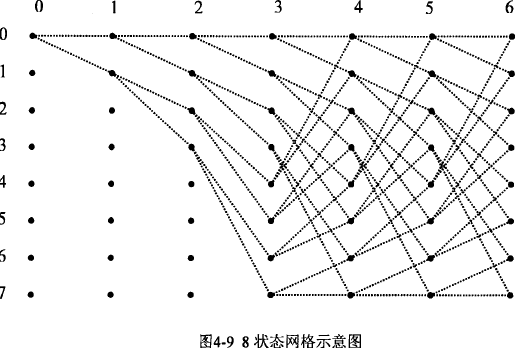图4.10是当L=2时局部优选路径的比较选择示意图。当t=4时，每个状态都有两条路径进入，每个状态需要保存两条局部优选路径度量以及相应的路径(局部最优路径，局部次优路径)。当t=5时，每一个状态都有四条路径进入，在这4条路径中通过比较选择得到局部最优路径和局部次优路径。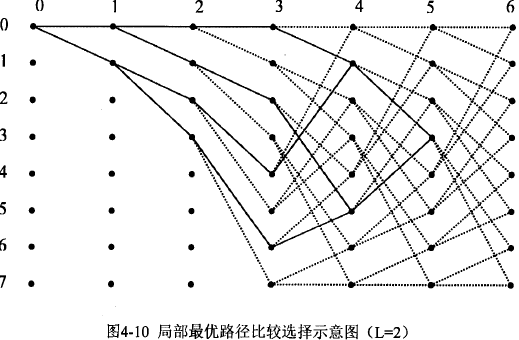图4.11是当L=4时局部优选路径的比较选择示意图。当t=5时，对于每一个状态都有4条路径进入，每个状态需要保存4条局部优选路径度量以及相应的路径(局部最优路径，局部次优路径，局部第3优路径，局部第4优路径)。当t=6时，每一个状态都有8条路径进入，此时需要在这8条路径中比较选择得到4条局部优选路径。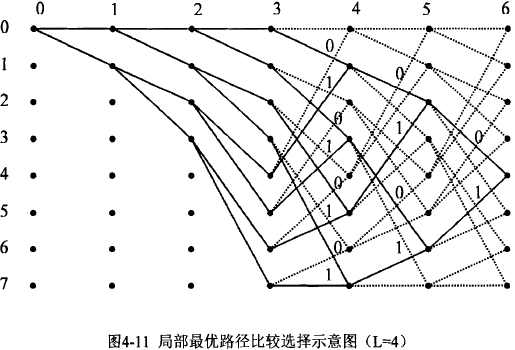如上图4.11所示，当t=6时，对于状态4而言，总共有8条路径到达该状态，当前状态的局部路径度量（Path Metric）分别表示为：PM10、PM20、PM30、PM40、PM11、PM21、PM31、PM41。PM10表示由上个状态的第一条最优路径经0输入得到当前状态路径度量。PM20表示由上个状态的第二条最优路径经0输入得到当前状态路径度量。BM0表示由当前状态经0回溯的分支度量（Branch Metric）。
PM10=PM（1，input0）+BM0；
PM20=PM（2，input0）+BM0；
PM30=PM（3，input0）+BM0；
PM40=PM（4，input0）+BM0；

PM11=PM（1，input1）+BM1；
PM21=PM（2，input1）+BM1；
PM31=PM（3，input1）+BM1；
PM41=PM（4，input1）+BM1；
在选取的4条局部优选路径时，注意到当前状态的局部路径度量之间必然有以下关系：PM10>PM20>PM30>PM40；PM11>PM21>PM31>PM41。两组之间关系不确定，利用以上的不等式关系，得PLVA算法中局部优选路径的选择判断步骤(这里的描述包括L=1／2／3／4的情形，其它情形可以依次类推)：

1)求当前状态的局部最优路径度量(L=1)： PM（1，nowstate）=max(PM10，PM11)；
2)求当前状态的局部次优路径度量(L=2)
if (L=1：PM10)，则PM（2，nowstate）=max(PM11，PM20)；
if (L=1：PM11)，则PM（2，nowstate）=max(PM10，PM21)；

3)求当前状态的第三路径度量(L=3)
if (L=2：PM10，PM11)，则PM（3，nowstate）=max(PM20，PM21)；
if (L=2：PM10，PM20)，则PM（3，nowstate）=max(PM11，PM30)；
if (L=2：PM11，PM10)，则PM（3，nowstate）=max(PM21，PM20)；
if (L=2：PM11，PM21)，则PM（3，nowstate）=max(PM31，PM10)；
4)求当前状态的第四路径度量(L=4) if (L=3：PM10，PM11，PM20)，则PM（4，nowstate）=max(PM21，PM30)；
if (L=3：PM10，PM11，PM21)，则PM（4，nowstate）=max(PM20，PM31)；
if (L=3：PM10，PM20，PM11)，则PM（4，nowstate）=max(PM21，PM30)；
if (L=3：PM10，PM20，PM30)，则PM（4，nowstate）=max(PM40，PM11)；
if (L=3：PM11，PM10，PM21)，则PM（4，nowstate）=max(PM20，PM31)；
if (L=3：PM11，PM10，PM20)，则PM（4，nowstate）=max(PM21，PM30)；
if (L=3：PM11，PM21，PM31)，则PM（4，nowstate）=max(PM10，PM41)；
if (L=3：PM11，PM21，PM10)，则PM（4，nowstate）=max(PM20，PM31)；
从上面的选择过程可以看出，当L=4时，对于每一个状态的选取4条优选路径，实际只需要进行4次比较即可。
3.2 全局优选路径的回溯过程
对于L=4的PLVA算法，对于每个时刻的每一个状态都有相应的路径寄存器Path_reg来存储路径信息，在Path _reg保存有4个值，可以得到前一时刻的相应状态，Path_reg[0/1/2/3]按照从左到右的顺序，依次存储[最优路径/次优路径/第3优路径/第4优路径的路径状态信息]，回溯即可得到完整的路径以及相应的输出。回溯网格如图4-12。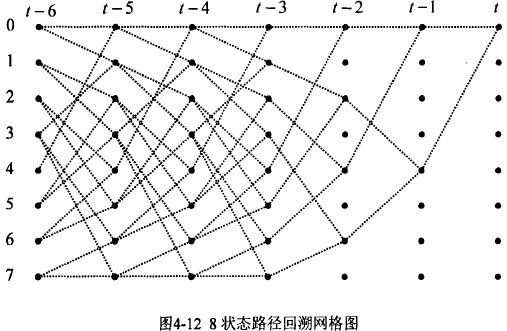图4.13给出PLVA译码当L=4时的路径回溯过程示意图。在回溯过程中，4条优选路径首先从占据的0状态开始，以后可能会占据某一时刻的1、2、3或者4个状态，注意这4条优选路径最多只可能占据某一时刻的4个状态。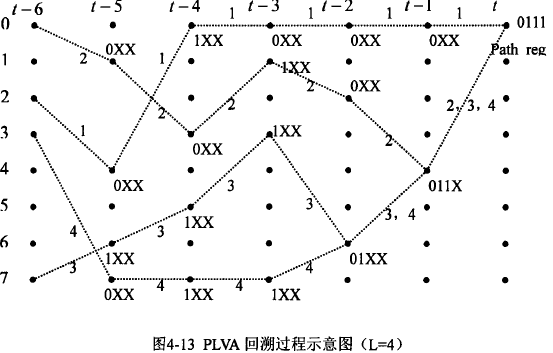展开全文• 针对 Turbo码译码器功耗大的问题,改进了传统的最大后验概率译码算法,提出了一种基于网格图 合并思想的低功耗 Turbo译码算法 .该算法通过减少计算过程中占功耗绝大部分的存储器的访问次数来 达到降低译码功耗的目的...
• 在通信系统中使用了MAP算法的Turbo译码算法
• 探讨基于Turbo原理进行迭代检测的编码连续相位调制（CPM）系统在无限瑞利衰落信道下的Log-MAP译码算法。根据CPM的分解模型，CPM分解为连续相位编码（CPE）与无记忆调制（MM）的组合。基于CPE的记忆特性和递归特性，...
• 本文主要介绍了短帧Turbo译码器的FPGA实现，并对相关参数和译码结构进行了描述。1几种译码算法比较Turbo码常见的几种译码算法中，MAP算法具有最优的译码性能。但因其运算过程中有较多的乘法和指数运算，硬件...FPGA
• Turbo译码器中的分量译码器的实现算法有SOVA算法，Max-Log-Map算法和Log-Map算法，其中SOVA算法复杂度最低，性能最差；Log-Map算法性能最佳，复杂度最大，本文采用基于Max-Log-Map的优化译码算法，对状态量度归一化...
• Turbo译码算法的研究与改进，周海霞，魏洪涛，如今社会早已进入信息时代，人们对通信质量的要求不断提高，对通信技术的关注也越来越密切。现在4G已经进入人们的生活，人们能够�通信技术
• Turbo码的译码算法大致可分为串行译码算法和并行译码算法两大类。串行译码算法如MAP、LOG- MAP等的研究已比较深入。但并行译码算法,尚有许多问题有待探讨。研究了Turbo码的并行译码算法,将Turbo码译码和图论结合起来...
• 获得优良的差错控制特性和多次迭代处理产生大的时间延迟是一对矛盾。为了有效解决这一问题，对Turbo码机理和迭代译码...计算机仿真以及对仿真结果的比较分析证明了这种改进的Turbo译码算法能够有效减少译码时间延迟。
• 针对传统的Max-Log-Map译码算法时效性差、存储空间开销大的特点，本文对传统的Max-Log-Map译码算法进行了改进。改进的算法对前、后向度量使用了蝶形结构图，便于DSP实现；将原始帧均分为多个子块，设计子块间的并行...
• 研究了分组相关快衰落信道的特性，推导出该信道下Turbo码的译码算法，并从推导结果确定：平均信噪比和信噪比弥散度是影响系统性能的两个重要参数。仿真结果表明，在分组相关快衰落信道下，采用该文提出的Turbo译码...
• 基于MATLAB的Turbo译码算法研究.pdf
• LogMAP算法是Turbo译码算法的一种简化算法，这类算法仍具有译码复杂度高，译码时延大的缺点。针对这一问题，提出了一种简化的对数最大后验概率译码算法。该算法基于逼近理论，用分段式最佳平方逼近多项式近似...最佳平方逼近
• 针对分组Turbo码自适应Chase译码算法中SNR是预先设定值的情况，提出一种新的自适应估计信噪比（SNR）的译码算法。该方案利用了接收码字的统计信息与SNR之间存在的对应关系来调整门限函数，达到控制译码复杂度的目的...
• 基于MATLAB的Turbo译码算法研究分析.pdf
• 纠错码中turbo码的译码原理 MAP算法。自己写，照下来的图片格式。参考王新梅的纠错码一书
• 一种改进的Turbo译码算法及其FPGA实现.pdf
• 针对由扩展汉明码构建的分组Turb0码，提出了一种可行的估计无竞争码字比特外部信息值的取值方法，并与现有文献中的方法进行仿真比较.仿真结果表明，该方法在高斯信道和Rayleigh衰落信道中都得到较好的译码性能.
• map算法MAP算法在Turbo码译码中的实现及性能在数域中，串行级联的MAP算法是用于获得高性能的Turbo码译码器。一般情况下，解码器通过可编程门...随着Turbo码解码器同步技术的实现，提出了一种高效连续的MAP译码算法...